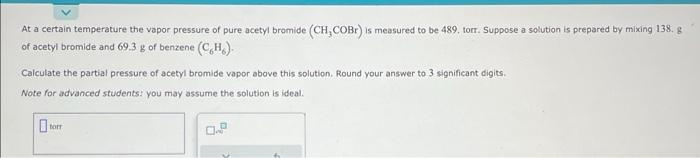Home / Expert Answers / Chemistry / at-a-certain-temperature-the-vapor-pressure-of-pure-acetyl-bramide-left-mathrm-ch-3-mathrm-pa786

# (Solved): At a certain temperature the vapor pressure of pure acetyl bramide $$\left(\mathrm{CH}_{3} \mathrm ...At a certain temperature the vapor pressure of pure acetyl bramide \( \left(\mathrm{CH}_{3} \mathrm{COBr}\right)$$ is measured to be 489 , torr. Suppose a solution is prepared by mixing $$138.8$$ of acetyl bromide and $$69.3 \mathrm{~g}$$ of benzene $$\left(\mathrm{C}_{6} \mathrm{H}_{6}\right)$$. Calculate the partial pressure of acetyl bromide vapor above this solution. Round your answer to 3 significant digits. Note for advanced students: you may assume the solution is ideal.

We have an Answer from Expert